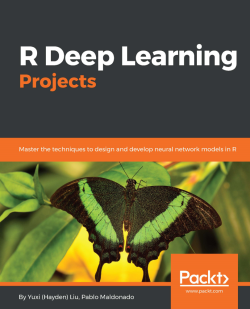# R Deep Learning Projects5 (1 reviews total)
By Yuxi (Hayden) Liu , Pablo Maldonado
What do you get with a Packt Subscription?

• Constantly updated with 100+ new titles each month
• Breadth and depth in over 1,000+ technologies

R is a popular programming language used by statisticians and mathematicians for statistical analysis, and is popularly used for deep learning. Deep Learning, as we all know, is one of the trending topics today, and is finding practical applications in a lot of domains.

This book demonstrates end-to-end implementations of five real-world projects on popular topics in deep learning such as handwritten digit recognition, traffic light detection, fraud detection, text generation, and sentiment analysis. You'll learn how to train effective neural networks in R—including convolutional neural networks, recurrent neural networks, and LSTMs—and apply them in practical scenarios. The book also highlights how neural networks can be trained using GPU capabilities. You will use popular R libraries and packages—such as MXNetR, H2O, deepnet, and more—to implement the projects.

By the end of this book, you will have a better understanding of deep learning concepts and techniques and how to use them in a practical setting.

Publication date:
February 2018
Publisher
Packt
Pages
258
ISBN
9781788478403

## Chapter 1. Handwritten Digit Recognition Using Convolutional Neural Networks

We kick off our R deep learning journey with the fundamental and core concepts of deep learning, and a deep learning 101 project—handwritten digit recognition. We will start with what deep learning is about, why we need it, and its evolution in recent years. We will also discuss why deep learning stands out and several typical deep learning applications. With the important deep learning concepts in mind, we get it started with our image classification project where we first conduct exploratory analysis on the data and make an initial attempt using shallow single-layer neural networks. Then we move on with deeper neural networks and achieve better results. However, we argue that chaining more hidden layers does not necessarily improve classification performance. The key is to extract richer representation and more informative features. And convolutional neural networks (CNNs) are the way to go! We will be demonstrating how we boost the digit recognition accuracy to nearly 99% with CNNs, which are well suited to exploiting strong and unique features that differentiate between images. We finally wrap up the chapter after several more experiments and validations.

We will look into these topics in detail:

• What is deep learning and what is special about it
• Applications of deep learning
• Exploratory analysis on MNIST handwritten digit data
• Handwritten digit recognition using logistic regression and single-layer neural networks with the `nnet` package
• Handwritten digit recognition using deep neural networks with the `MXNet` package
• Rectified linear unit
• The mechanics and structure of convolutional neural networks
• Handwritten digit recognition using convolutional neural networks with the `MXNet` package
• Visualization of outputs of convolutional layers
• Early stopping in deep neural networks

## What is deep learning and why do we need it?

Deep learning is an emerging subfield of machine learning. It employs artificial neural network (ANN) algorithms to process data, derive patterns or to develop abstractions, simulating the thinking process of a biological brain. And those ANNs usually contain more than one hidden layer, which is how deep learning got its name—machine learning with stacked neural networks. Going beyond shallow ANNs (usually with only one hidden layer), a deep learning model with the right architectures and parameters can better represent complex non-linear relationships.

Here is an example of a shallow ANN: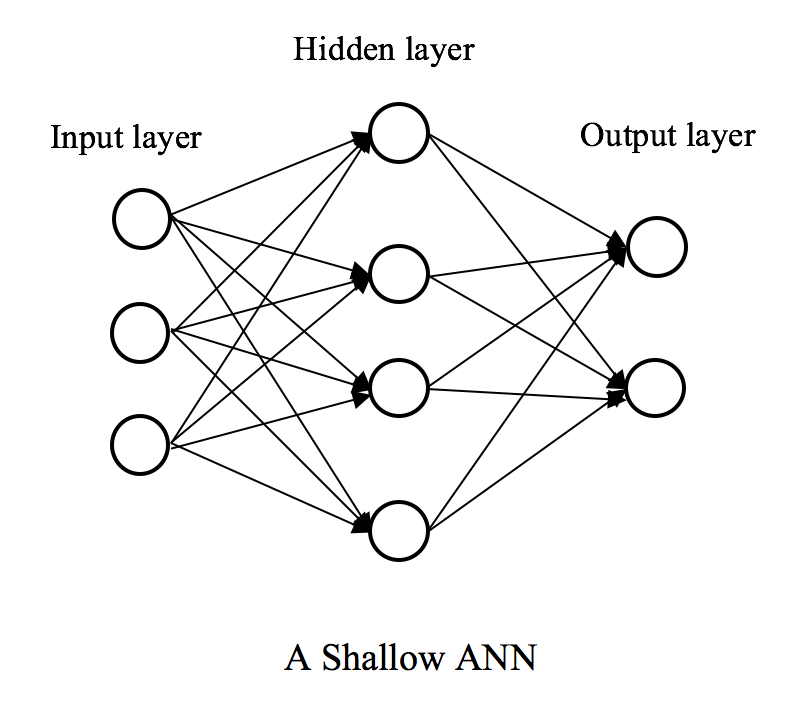And an example of a deep learning model: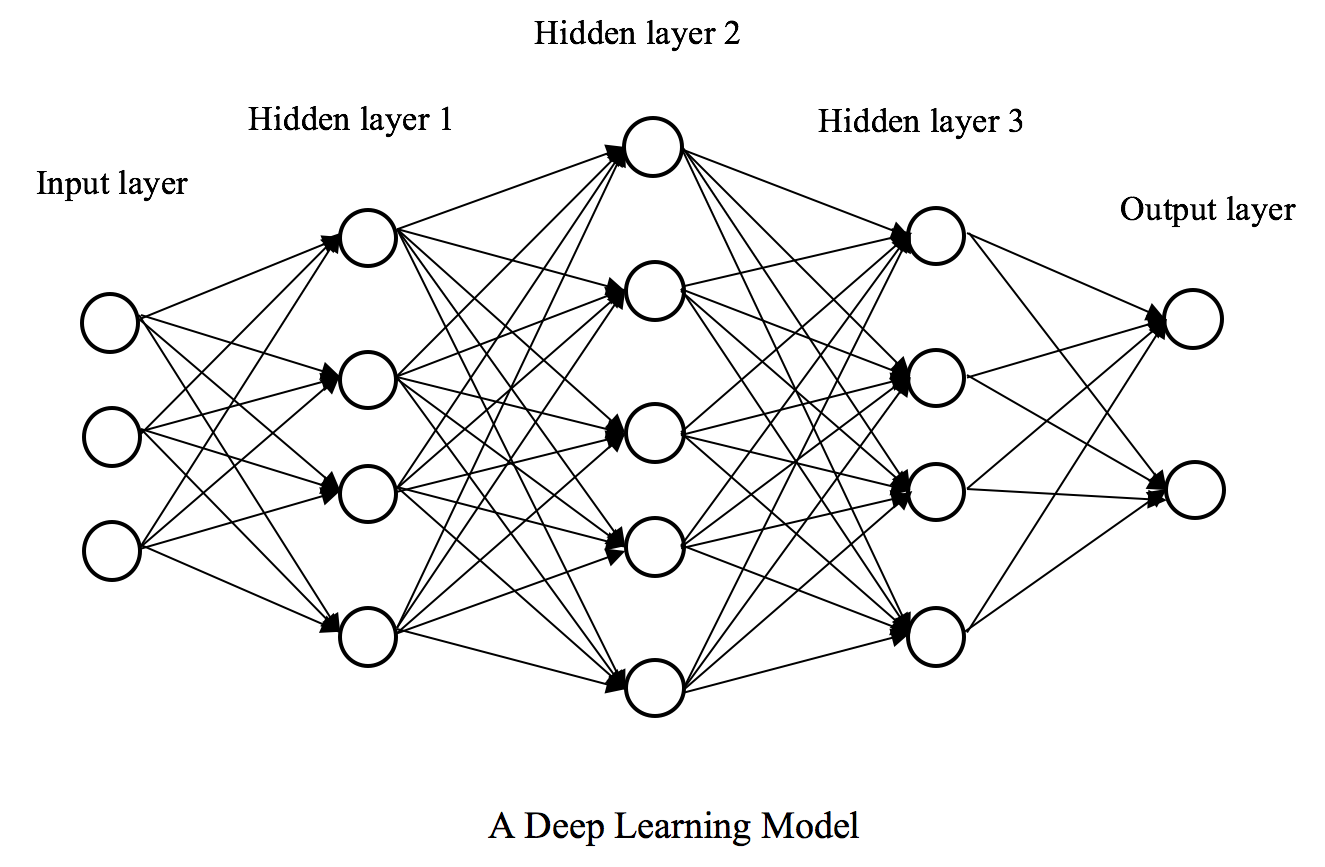Don't feel scared, regardless of how complicated it might sound or look. We will be going from shallow to deep dives into deep learning throughout five projects in this book.

First of all, as a part of the broad family of machine learning, deep learning can be used in supervised learning, semi-supervised learning, as well as unsupervised learning tasks, even reinforcement learning tasks. So what sets it apart from traditional machine learning algorithms?

### What makes deep learning special?

Deep learning employs a stack of multiple hidden layers of non-linear processing units. The input of a hidden layer is the output of its previous layer. This can be easily observed from the examples of a shallow neural network and a deep neural network shown previously.

Features are extracted from each hidden layer. Features from different layers represent abstracts or patterns of different levels. Hence, higher-level features are derived from lower-level features, which are extracted from previous layers. All these together form a hierarchical representation learned from the data.

Take the cats and dogs image classification as an example, in traditional machine learning solutions, the classification step follows a feature extraction process, which is often based on:

• Domain knowledge, such as color, shape, color of the animals, shape of the ears in this case, which are usually hand-crafted
• Dimensionality reduction, such as principal component analysis (PCA), Latent Dirichlet Allocation (LDA)
• Feature engineering techniques, such as histogram of oriented gradients transformation (HOG), Scale Invariant Feature Transform (SIFT), and Speeded up Robust Features (SURF)

The workflow of traditional machine learning solution to cats and dogs classification is displayed as follows: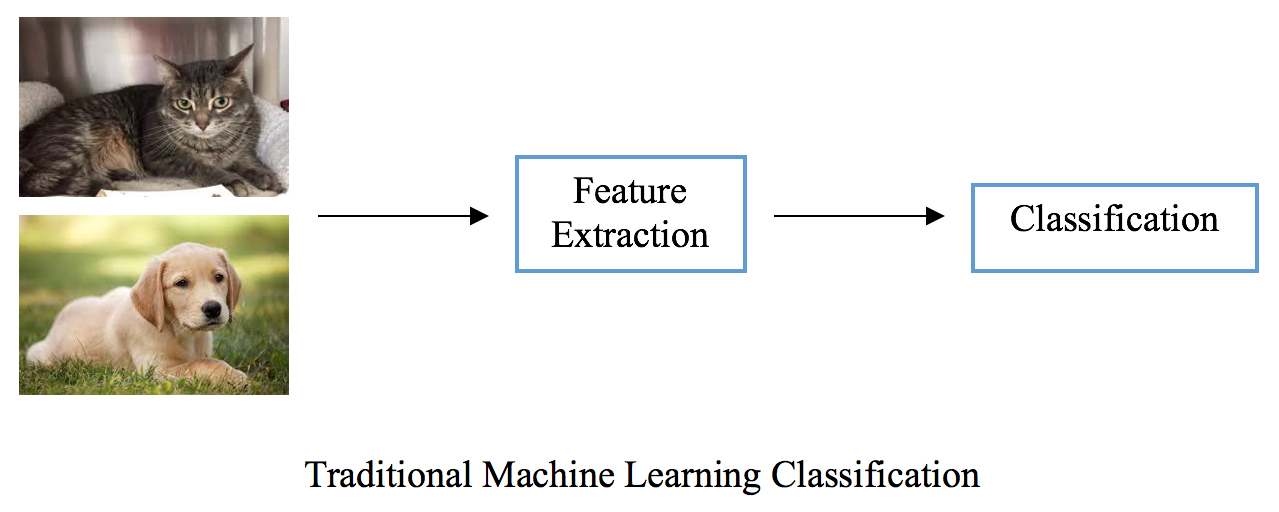However, in deep learning based solutions (such as CNNs, which we will be learning shortly), hierarchical representations are derived throughout the latent learning process and features of the highest level are then fed into the final classification step. These features capture the important and distinguishable details in the cat and dog images. Depending on the magic worked in hidden layers:

• The low-level features can be edges, lines or dots of whiskers, nose or eyes, ears and so on
• The higher-level features can be outlines or contours of the animals

The entire workflow of deep learning solution is shown as follows: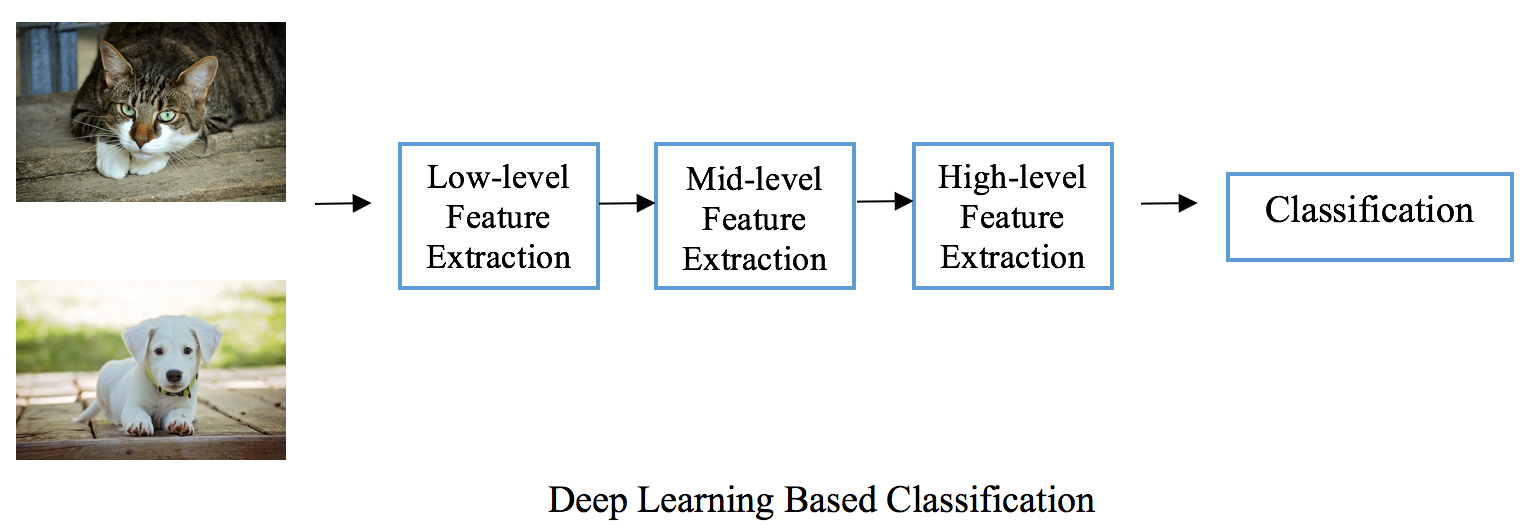Deep learning removes those manual or explicit feature extraction steps, and instead relies on the training process to automatically discover useful patterns underneath the input data. And through tweaking the layout (number of layers, number of hidden units for a layer, activation function, and so on) of the networks, we can find the most efficient sets of features.

Recall the example of the shallow ANN and that of the deep learning model in the last section, data flow one-way from the input layer to the output. Besides feedforward architectures, deep learning models allow data to proceed in any direction, even to circle back to the input layer. Data looping back from the previous output becomes part of the next input data. Recurrent neural networks (RNNs) are great examples. We will be working on projects using RNNs later in this book. For now, we can still get a sense of what the recurrent or cycle-like architecture looks like from the diagram of RNNs as follows: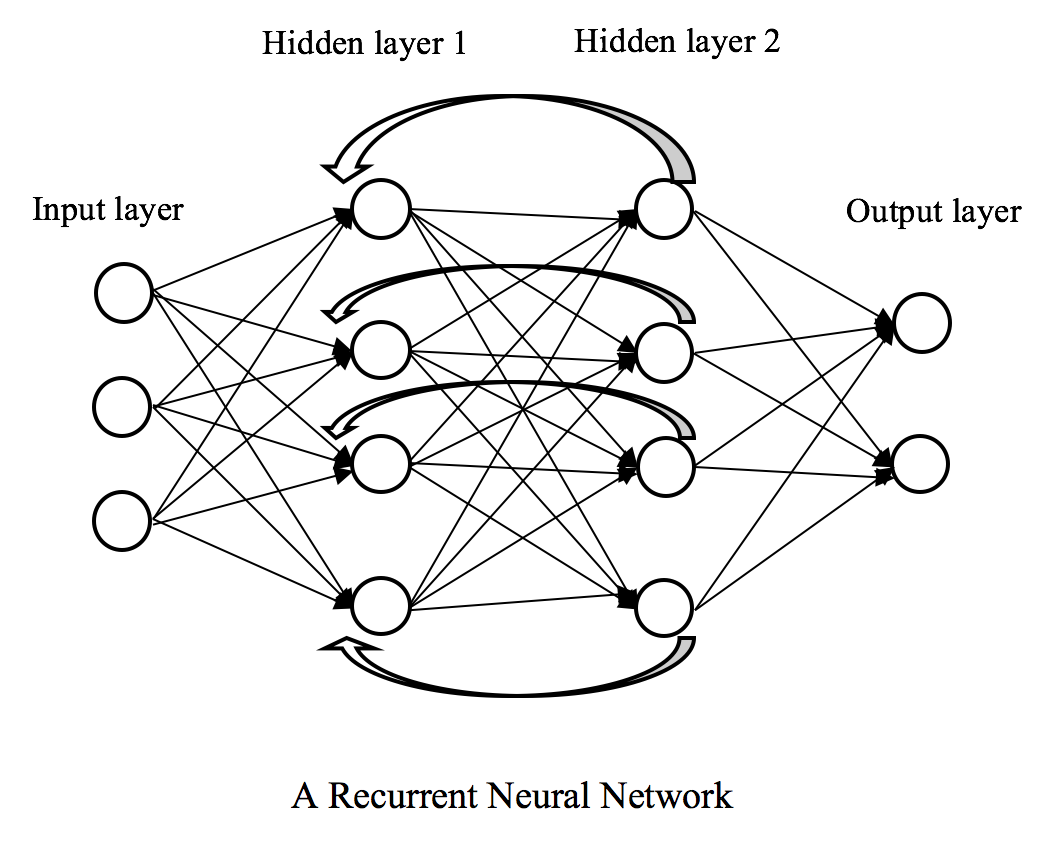The recurrent architecture makes the models applicable to time series data and sequences of inputs. As data from previous time points goes into the training of the current time point, the deep learning recurrent model effectively solves a time series or sequence learning problem in a feedforward manner. In traditional machine learning solutions (read more in Machine Learning for Sequential Data: A Review by T. Dietterich) to time series problems, sliding windows of previous lags are usually provided as current inputs. This can be ineffective as the size of the sliding windows needs to be decided and so does the number of windows, while the recurrent models figure out timely or sequential relationships themselves.

### Note

Although we are discussing here all the advantages about deep learning over the other machine learning techniques, we did not make any claim or statement that the modern deep learning is superior to the traditional machine learning. That's right, there is no free lunch in this field, which was also emphasized in my last book, Python Machine Learning By Example. There is no single algorithm that can solve all machine learning problems more efficiently than others. It all depends on specific use cases - in some applications, the "traditional" ones are a better fit, or a deep learning setting makes no difference; in some cases, the "modern" ones yield better performance.

Next, we will see some typical applications of deep learning that will better motivate us to get started in deep learning projects.

### What are the applications of deep learning?

Computer vision and image recognition is often considered the first area where breakthroughs of deep learning occurred. Handwritten digit recognition has become a Hello World in this field, and a common evaluation set for image classification algorithms and techniques is the scanned document dataset constructed from the National Institute of Standards and Technology (NIST), called MNIST (M stands for modified, which means data is pre-processed for the ease of machine learning processes).

Some examples from MNIST are shown as follows: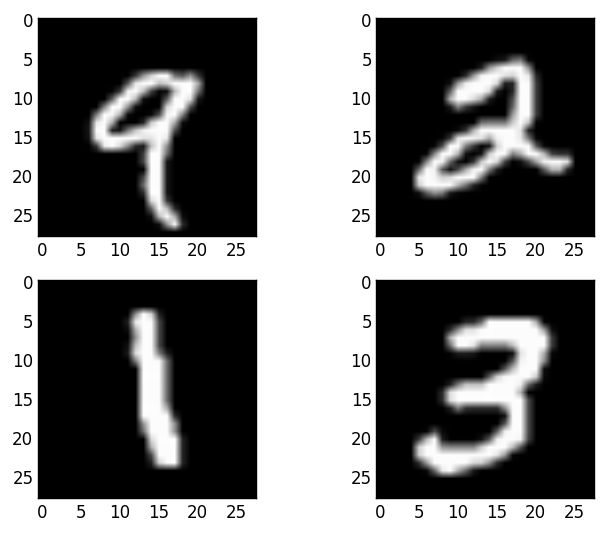Some researchers have so far achieved the best performance 0.21% error rate on the MNIST dataset using CNNs. Details can be found in the paper, Regularization of Neural Networks using DropConnect, published in the International Conference on Machine Learning (ICML) in 2013. Other comparable results, for example 0.23%, 0.27% and 0.31%, are also yielded by CNNs and deep neural networks. However, traditional machine learning algorithms with sophisticated feature engineering techniques could only yield error rates ranging from 0.52% to 7.6%, which were achieved by using Support Vector Machine (SVMs) and pairwise linear classifiers respectively.

Besides image recognition (such as the well known face recognition), the applications of deep learning are extended to more challenging tasks including:

• Image-based search engines, which cover image classification and image similarity encoding, heavily utilizing deep learning techniques.
• Machine vision, with self-driving cars as an example, which interprets 360° camera views to make decisions in real time.
• Color restoration from black and white photos—the examples after color recovery from http://hi.cs.waseda.ac.jp/~iizuka/projects/colorization/extra.html are impressive.
• Image generation, including handwriting, cat images, and even video game images, or whatever image you name it. For example, we use an interesting playground, https://www.cs.toronto.edu/~graves/handwriting.html (developed by Alex Graves from the University of Toronto), to create handwritings of the title of this book in three different styles: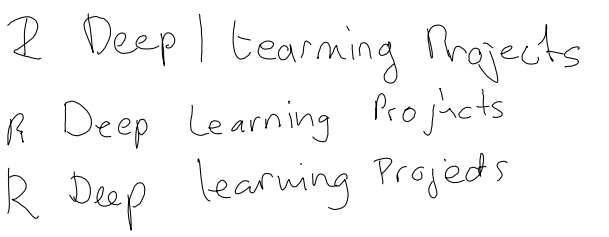Natural language processing (NLP) is another field where deep learning is dominant in modern solutions. Recall we described deep learning models with recurrent architecture are appropriate for sequences of inputs, such as natural language and text. In recent years, deep learning has greatly helped to improve:

• Machine translation, for example the sentence-based Google Neural Machine Translation system (GNMT) which utilizes deep RNNs to improve accuracy and fluency
• Sentiment analysis, information retrieval, theme detection and many other common NLP applications, where deep learning models have achieved state-of-the-art performance thanks to word embedding techniques
• Text generation, where RNNs learn the intricate relationship between words (including punctuation) in sentences and to write text, to become an author or a virtual Shakespeare

Image captioning generation, also known as image to text, couples recent breakthroughs in computer vision and NLP. It leverages CNNs to detect and classify objects in images, and assigns labels to those objects. It then applies RNNs to describe those labels in a comprehensible sentence. The following examples are captured from the web demo from http://cs.stanford.edu/people/karpathy/deepimagesent/generationdemo/ (developed by Andrej Karpathy from Stanford University):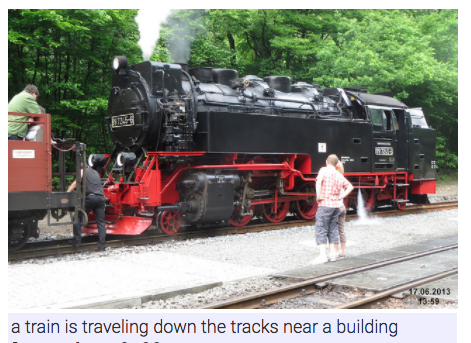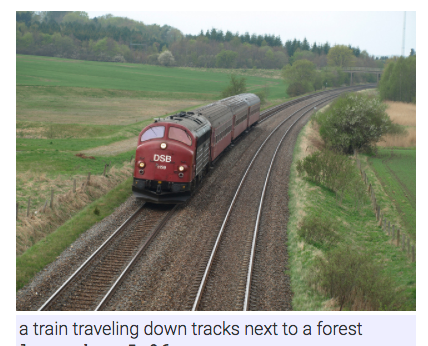Similarly, sound and speech is also a field of sequential learning, where machine learning algorithms are applied to predict time series or label sequence data. Speech recognition has been greatly revolutionized by deep learning. And now, deep learning based products like Apple's Siri, Amazon's Alexa, Google Home, Skype Translator and many others are "invading" our lives, in a good way for sure. Besides an author writing text, deep learning models can also be a music composer. For example, Francesco Marchesani from the Polytechnic University of Milan was able to train RNNs to produce Chopin's music.

Additionally, deep learning also excels in many use cases in video. It makes significant contributions to the boost of virtual reality with its capability of accurate motion detection, and to the advance of real-time behavior analysis in surveillance videos. Scientists from Google, DeepMind, and Oxford even built a computer lip reader called LipNet, achieving a success rate of 93%.

Besides supervised and unsupervised learning cases, deep learning is heavily used in reinforcement learning. Robots who can handle objects, climb stairs, operate in kitchens are not new to us. Recently, Google's AlphaGo beating the world's elite Go players received widespread media coverage. Nowadays, everybody looks forward to seeing self-driving cars being out in the market in just one or two years. These have all benefited from the advance of deep learning in reinforcement learning. Oh, and don't forget computers are taught to play the game, FlappyBird!

We did not even mention bioinformatics, drug discovery, recommendation systems in e-commerce, finance, especially the stock market, insurance and the Internet of Things (IoT). In fact, the list of deep learning applications is already long, and only gets longer and longer.

I hope this section excited you about deep learning and its power of providing better solutions to many machine learning problems we are facing. Artificial intelligence has a brighter future thanks to the advance of deep learning.

So what are we waiting for? Let's get started with handwritten digit recognition!

## Handwritten digit recognition using CNNs

For sure, we begin with exploration of the handwritten digit dataset.

### Get started with exploring MNIST

The MNIST dataset from http://yann.lecun.com/exdb/mnist/ consists of a training set of 60,000 samples, and a testing set of 10,000 samples. As said previously, images were originally taken from the NIST, and then centered and resized to the same height and width (28 * 28).

Rather than handling the `ubyte` files, `train-images-idx3-ubyte.gz` and `train-labels-idx1-ubyte.gz` in the preceding website and merge them, we use a dataset that is well-formatted from the Kaggle competition Digit Recognizer, https://www.kaggle.com/c/digit-recognizer/. We can download the training dataset, `train.csv` directly from https://www.kaggle.com/c/digit-recognizer/data. It is the only labeled dataset provided in the site, and we will use it to train classification models, evaluate models and do predictions. Now let's load it up:

```> data <- read.csv ("train.csv")
> dim(data)
 42000 785```

We have 42,000 labeled samples available, and each sample has 784 features, which means each digit image has 784 (28 * 28) pixels. Take a look at the label and the first 5 features (pixels) for each of the first 6 data samples:

```> head(data[1:6])
label pixel0 pixel1 pixel2 pixel3 pixel4
1 1 0 0 0 0 0
2 0 0 0 0 0 0
3 1 0 0 0 0 0
4 4 0 0 0 0 0
5 0 0 0 0 0 0
6 0 0 0 0 0 0```

The target label ranging from `0` to `9` denotes 10 digits:

```> unique(unlist(data))
 1 0 4 7 3 5 8 9 2 6```

The pixel variable ranges from `0` to `255`, representing the brightness of the pixel, for example `0` means black and `255` stands for white:

```> min(data[2:785])
 0
> max(data[2:785])
 255```

Now let's take a look at two samples, first, the fourth image:

```> sample_4 <- matrix(as.numeric(data[4,-1]), nrow = 28, byrow = TRUE)
> image(sample_4, col = grey.colors(255))```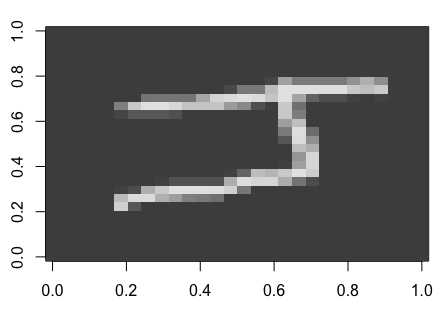Where we reshaped the feature vector of length 784 into a matrix of 28 * 28.

Second, the 7th image:

```> sample_7 <- matrix(as.numeric(data[7,-1]), nrow = 28, byrow = TRUE)
> image(sample_7, col = grey.colors(255))```

The result is as follows: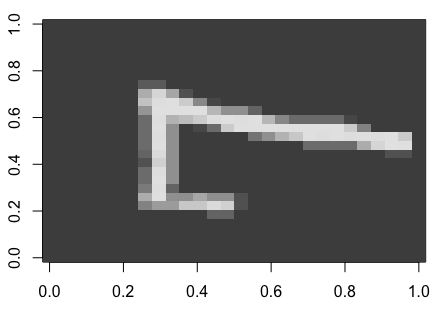We noticed that the images are rotated 90 degrees to the left. To better view the images, a rotation of 90 degrees clockwise is required. We simply need to reserve elements in each column of an image matrix:

```> # Rotate the matrix by reversing elements in each column
> rotate <- function(x) t(apply(x, 2, rev))```

Now visualize the rotated images:

`> image(rotate(sample_4), col = grey.colors(255))`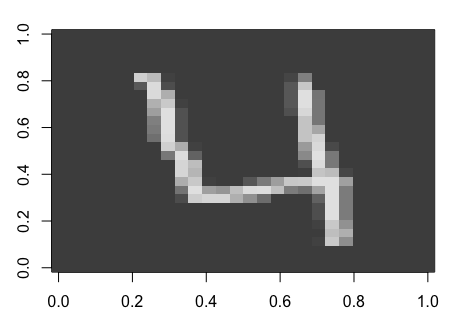`> image(rotate(sample_7), col = grey.colors(255))`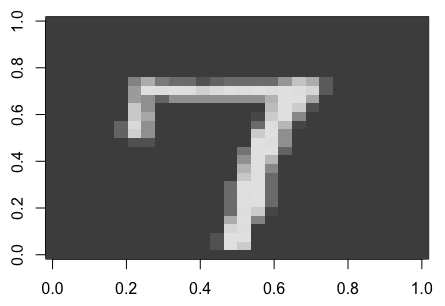After viewing what the data and images behind look like, we do more exploratory analysis on the labels and features. Firstly, because it is a classification problem, we inspect whether the classes from the data are balanced or unbalanced as a good practice. But before doing so, we should transform the label from integer to factor:

```> # Transform target variable "label" from integer to factor, in order to perform classification
> is.factor(data\$label)
 FALSE
> data\$label <- as.factor(data\$label)
> is.factor(data\$label)
 TRUE```

Now, we can summarize the label distribution in counts:

```> summary(data\$label)
0 1 2 3 4 5 6 7 8 9
4132 4684 4177 4351 4072 3795 4137 4401 4063 4188```

Or combined with proportion (%):

```> proportion <- prop.table(table(data\$label)) * 100
> cbind(count=table(data\$label), proportion=proportion)
count proportion
0 4132 9.838095
1 4684 11.152381
2 4177 9.945238
3 4351 10.359524
4 4072 9.695238
5 3795 9.035714
6 4137 9.850000
7 4401 10.478571
8 4063 9.673810
9 4188 9.971429```

Classes are balanced.

Now, we explore the distribution of features, the pixels. As an example, we take the 4 pixels from the central 2*2 block (that is, `pixel376`, `pixel377`, `pixel404`, and `pixel405)` in each image and display the histogram for each of the 9 digits:

```> central_block <- c("pixel376", "pixel377", "pixel404", "pixel405")
> par(mfrow=c(2, 2))
> for(i in 1:9) {
+ hist(c(as.matrix(data[data\$label==i, central_block])),
+ main=sprintf("Histogram for digit %d", i),
+ xlab="Pixel value")
+ }```

The resulting pixel brightness histograms for digit `1` to `4` are displayed respectively, as follows: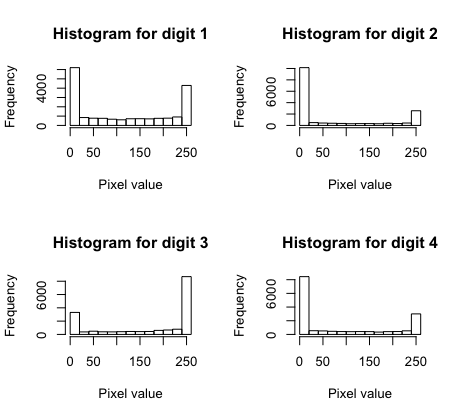Histograms for digits `5` to `9`: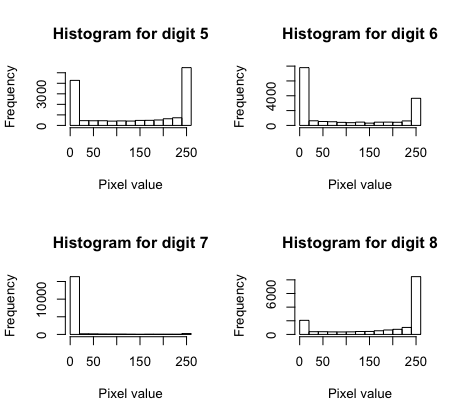And that for digit `9`: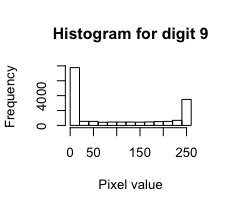The brightness of central pixels is distributed differently among `9` digits. For instance, the majority of the central pixels are bright, as digit `8` is usually written in a way that strokes go through the center; while digit `7` is not written in this way, hence most of the central pixels are dark. Pixels taken from other positions can also be distinctly distributed among different digits.

The exploratory analysis we just conducted helps move us forward with building classification models based on pixels.

### First attempt – logistic regression

We start off with probably the most basic classifier, the logistic regression, to be specific multinomial logistic regression as it is a multiclass case. It is a probabilistic linear classifier parameterized by a weight matrix W (also called coefficient matrix) and a bias (also called intercept) vector b. And it maps an input vector x to a set of probabilities P(y=1), P(y=2),. . ., P(y-K) for K possible classes.

A multinomial logistic regression for two possible classes can be represented graphically as follows: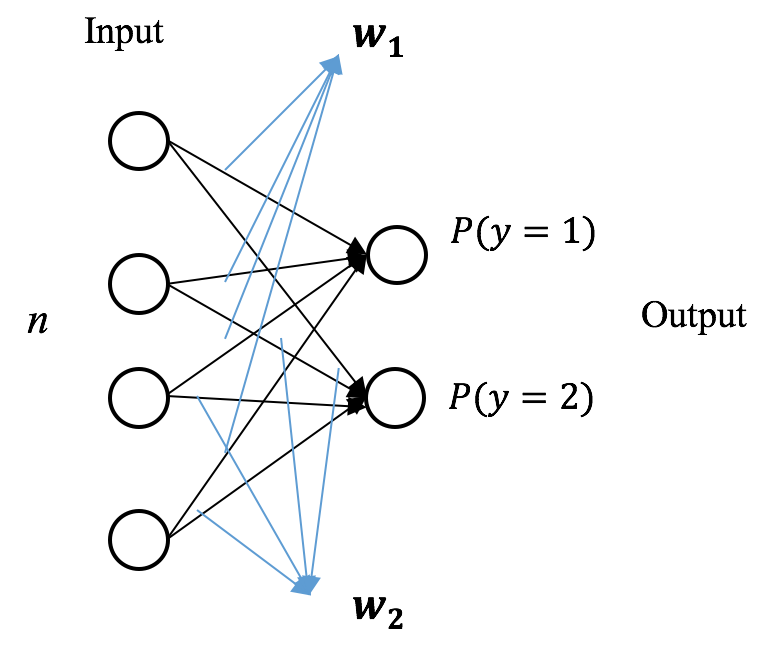Suppose x is n-dimension, then the weight matrix W is of size n by K with each column Wk representing the coefficients associated with class k; similarly, the bias vector b is of length K, with each element bk served as the bias for class k. For simplicity, the bias b can be viewed as an additional row in the weight matrix W. So the probability of x being class k can be expressed mathematically as:Where softmax() denotes the softmax function and that is why multinomial logistic regression is often called softmax regression.

Given a set of training sampleswhere, the optimal model w is obtained by minimizing the cost (also called log loss), which is defined as:whereAs usual we resort to gradient descent, an iterative optimization algorithm, to solve for the optimal w. In each iteration, w moves a step that is proportional to the negative derivative Δw of the objective function at the current point. That is, w:=w – ƞΔw, where ƞ is the learning rate. Each column Δwk  of Δw can be computed as:The well trained model, the optimal w will be used to classify a new sample x' by: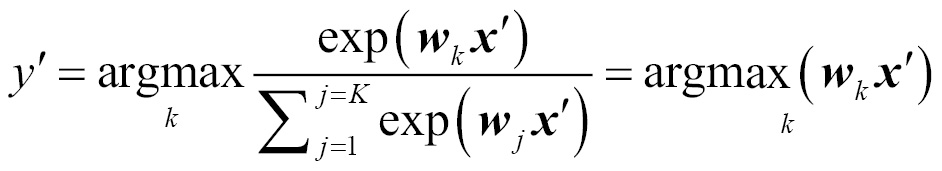Armed with the mechanics of the multinomial logistic regression we just reviewed, we can then apply it as the first solution to our digit classification project.

We first split the dataset into two subsets for training and testing respectively using the `caret` package.

### Note

caret stands for classification and regression training. The package is designed to facilitate the process for training and evaluating models. It contains tools and methods for data splitting, data pre-processing, feature selection and model tuning. Documentation and a full list of functions can be found in https://cran.r-project.org/web/packages/caret/caret.pdf.

Install and import package `caret`:

```> if (!require("caret"))
+ install.packages("caret")
> library (caret)

We first split the data into two partitions, 75% for training and 25% for testing, using the `createDataPartition` function:

```> set.seed(42)
> train_perc = 0.75
> train_index <- createDataPartition(data\$label, p=train_perc, list=FALSE)```
```> data_train <- data[train_index,]
> data_test <- data[-train_index,]```

### Note

To ensure the experiments are reproducible, it is always a good practice to pick a seed from the random number generator.

Then, we implement the multinomial logistic regression model using the `nnet` package. The package contains functions for feed-forward single-layer neural networks as well as multinomial logistic regression models. More details can be found in https://cran.r-project.org/web/packages/nnet/nnet.pdf:

```> library(nnet)
> # Multinomial logistic regression
> model_lr <- multinom(label ~ ., data=data_train, MaxNWts=10000,
decay=5e-3, maxit=100)
# weights: 7860 (7065 variable)
initial value 72538.338185
iter 10 value 17046.804658
iter 20 value 11166.225504
iter 30 value 9514.340319
iter 40 value 8819.724147
iter 50 value 8405.001712
iter 60 value 8164.997939
iter 70 value 7983.427139
iter 80 value 7897.005940
iter 90 value 7831.663204
iter 100 value 7730.047242
final value 7730.047242
stopped after 100 iterations```

We fit a multinomial logistic regression model on the training subset, with parameters which include:

• `MaxNWts=10000`: It allows, at most, 10,000 weights. In our case, there are (784 dimensions + 1 bias) * 10 classes = 7850 elements in the weight matrix w
• `decay=5e-3`: The regularization strength, the weight decay is 0.005
• `maxit=100`: The maximum number of iterations is set to be 100

The error value is printed for every 10 iterations, and it is decreasing. The model converges as the maximum number of iterations is reached. Then we use the trained model to predict the classes of the testing samples:

`> prediction_lr <- predict(model_lr, data_test, type = "class")`

Take a look at the prediction results of the first five samples:

```> prediction_lr[1:5]
 1 0 7 5 8
Levels: 0 1 2 3 4 5 6 7 8 9```

And their true values are:

```> data_test\$label[1:5]
 1 0 7 5 8
Levels: 0 1 2 3 4 5 6 7 8 9```

We can also obtain the confusion matrix by:

```> cm_lr = table(data_test\$label, prediction_lr)
> cm_lr```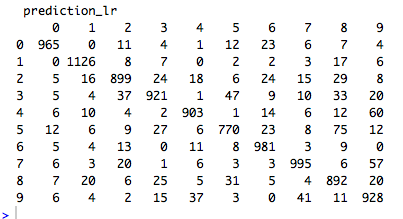And the classification accuracy:

```> accuracy_lr = mean(prediction_lr == data_test\$label)
> accuracy_lr
 0.8935886```

89.4% for the first try. Not bad! We could definitely do better by tweaking the model parameters, such as `decay` and `maxit`. But our focus is for a more advanced model that learns the underneath patterns better. So we move on with the second solution, the feed-forward neural networks with a single hidden layer.

### Going from logistic regression to single-layer neural networks

Basically, logistic regression is a feed-forward neural network without a hidden layer, where the output layer directly connects with the input layer. In other words, logistic regression is a single neuron that maps the input to the output layer. Theoretically, the neural networks with an additional hidden layer between the input and output layer should be able to learn more about the relationship underneath.

A single-layer neural network for two possible classes can be represented graphically as follows: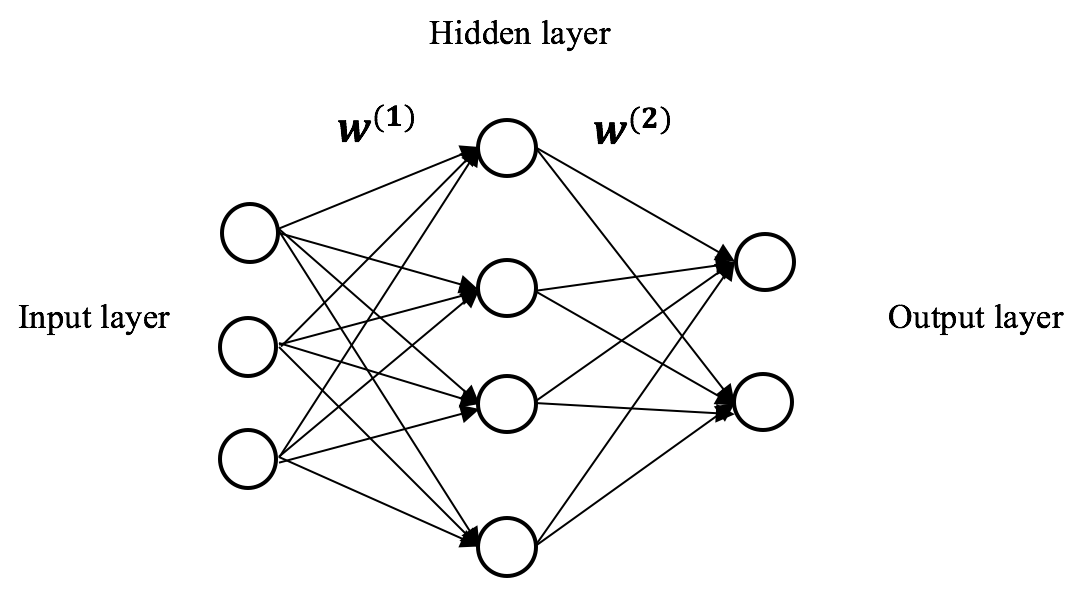Suppose x is n-dimension, and there are H hidden units in the hidden layer, then the weight matrix w(1) connecting the input layer to the hidden layer is of size n by H with each column wh(1) representing the coefficients associated with the h-th hidden unit. So, the output (also called activation) of the h-th hidden unit ah(2) can be expressed mathematically as:For example, for the outputs of the first, second and the last hidden unit:where f(z) is an activation function. Typical choices for the activation function in simple networks include logistic function (more often called sigmoid function) and `tanh` function (which can be considered a re-scaled version of logistic function):Plots of these two functions are as follows: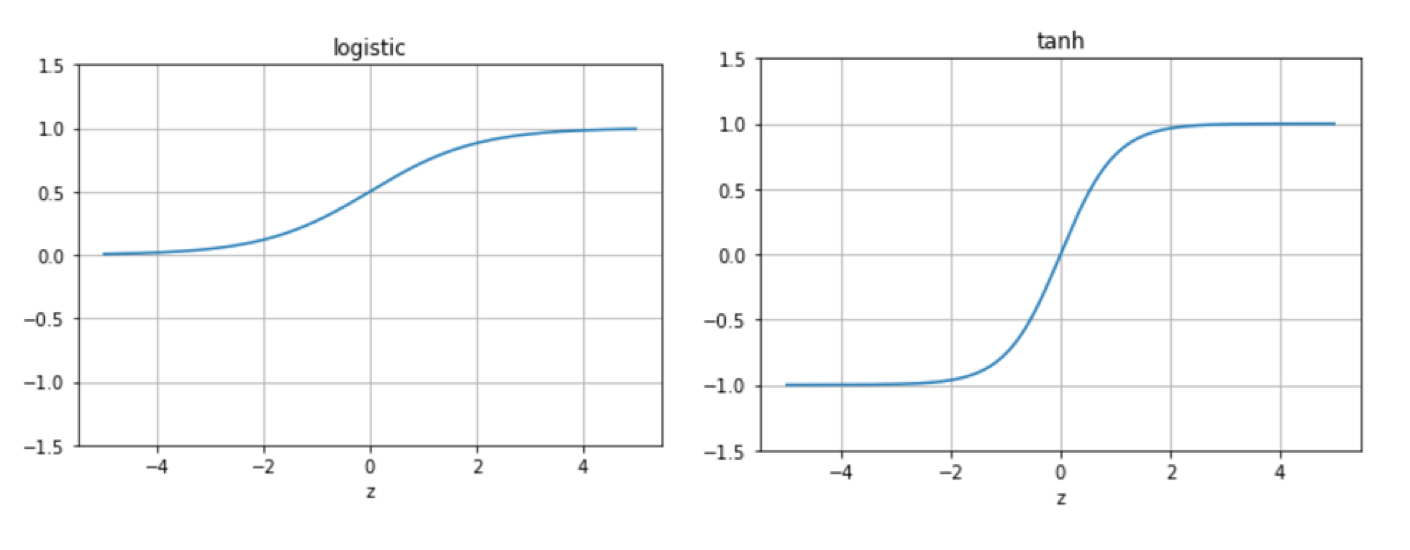We will be using the logistic function in our single-layered networks for now.

For a case of K possible classes, the weight matrix w(2) connecting the hidden layer to the output layer is of size H by K. Each column wk(2) represents the coefficients associated with class k. The input to the output layer is the output of the hidden layer a(2) = {a1(2), a2(2), ,...,aI(2) }, the probability of x being class k can be expressed mathematically as (for consistency, we denote it as ak(3)):Similarly, given m training samples, to train the neural network, we learn all weights w = {w(1)w(2)} using gradient descent with the goal of minimizing the mean squared error cost J(w).

Computation of the gradients Δw can be realized through the backpropagation algorithm. The idea of the backpropagation algorithm is the following, we first travel through the network and compute all outputs of the hidden layers and output layer; then moving backward from the last layer, we calculate how much each node contributed to the error in the final output and propagate it back to the previous layers. In our single-layer network, the detailed steps are:

1. Compute a(2) for the hidden layer and feed them to the output layer to compute the outputs a(3)
2. For the output layer, compute the derivative of the cost function of one sample j(W) with regards to each unit,, or, rewritten for the entire layer
3. For the hidden layer, we compute the error term δ(2) based on a weighted average of4. Compute the gradients applying the chain rule:We repeatedly update all weights by taking these steps until the cost function converges.

After a brief review of the single-layer network, we can then apply it as the second solution to our digit classification project.

Again, we use the `nnet` package to implement our single-layer network:

```> model_nn <- nnet(label ~ ., data=data_train, size=50, maxit=300, MaxNWts=100000, decay=1e-4)
# weights: 39760
initial value 108597.598656
iter 10 value 27708.286001
iter 20 value 16027.005297
iter 30 value 14058.141050
iter 40 value 12615.442747
iter 50 value 11793.700937
iter 60 value 11026.672273
iter 70 value 10654.855058
iter 80 value 10193.580947
iter 90 value 9854.836168
iter 100 value 9544.973159
iter 110 value 9307.192737
iter 120 value 9043.028253
iter 130 value 8845.069307
iter 140 value 8686.707561
iter 150 value 8525.104362
iter 160 value 8281.609223
iter 170 value 8140.051273
iter 180 value 7998.721024
iter 190 value 7854.388240
iter 200 value 7712.459027
iter 210 value 7636.945553
iter 220 value 7557.675909
iter 230 value 7449.854506
iter 240 value 7355.021651
iter 250 value 7259.186906
iter 260 value 7192.798089
iter 270 value 7055.027833
iter 280 value 6957.926522
iter 290 value 6866.641511
iter 300 value 6778.342997
final value 6778.342997
stopped after 300 iterations```

We fit the model with parameters including:

• `size=50`: There are 50 hidden units in the hidden layer.
• `MaxNWts=100000`: It allows at most 100,000 weights. In our case, there are (784 input dimensions + 1 bias) * 50 hidden units = 39,250 elements in the weight matrix w(1) and (50 hidden units + 1 bias) * 10 output units = 510 elements in the weight matrix w(1). So there are 39,760 weights in total.
• `decay=1e-4`: The regularization strength, the weight decay is `0.0001`.
• `maxit=300`: The maximum number of iterations is set to be 300.

We apply the trained network model on the testing set:

```> prediction_nn <- predict(model_nn, data_test, type = "class")
> cm_nn = table(data_test\$label, prediction_nn)
> cm_nn
prediction_nn
0 1 2 3 4 5 6 7 8 9
0 987 0 3 3 2 11 10 7 5 5
1 0 1134 9 6 0 2 0 5 11 4
2 14 9 918 31 11 11 7 15 22 6
3 3 1 17 966 0 41 3 14 24 18
4 4 3 8 2 929 4 11 11 5 41
5 12 2 6 17 5 851 15 9 26 5
6 10 1 14 0 9 14 970 0 15 1
7 4 4 23 6 5 2 0 1010 7 39
8 5 15 9 18 5 31 9 4 912 7
9 11 2 1 20 52 4 0 36 8 913
> accuracy_nn = mean(prediction_nn == data_test\$label)
> accuracy_nn
 0.9135944```

Better than our first attempt! Can we do better with deep learning models, say intuitively more hidden layers? Sure.

### Adding more hidden layers to the networks

We have just achieved 91.3% accuracy with a single-layer neural network model. Theoretically, we can obtain a better one with more than one hidden layer. As an example, we provide a solution of a deep neural network model with two hidden layers: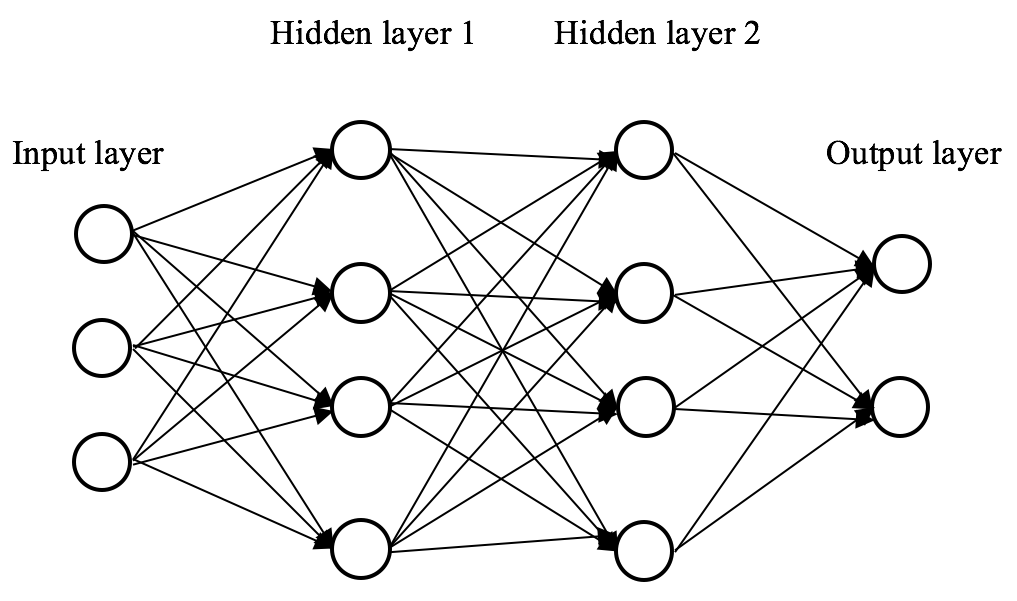Weight optimization in feed-forward deep neural networks is also realized through the backpropagation algorithm, which is identical to single-layer networks. However, the more layers, the higher the computation complexity, and the slower the model convergence. One way to accelerate the weight optimization, is to use a more computational efficient activation function. The most popular one in recent years is the rectified linear unit (ReLU):A plot of the ReLU function is as follows: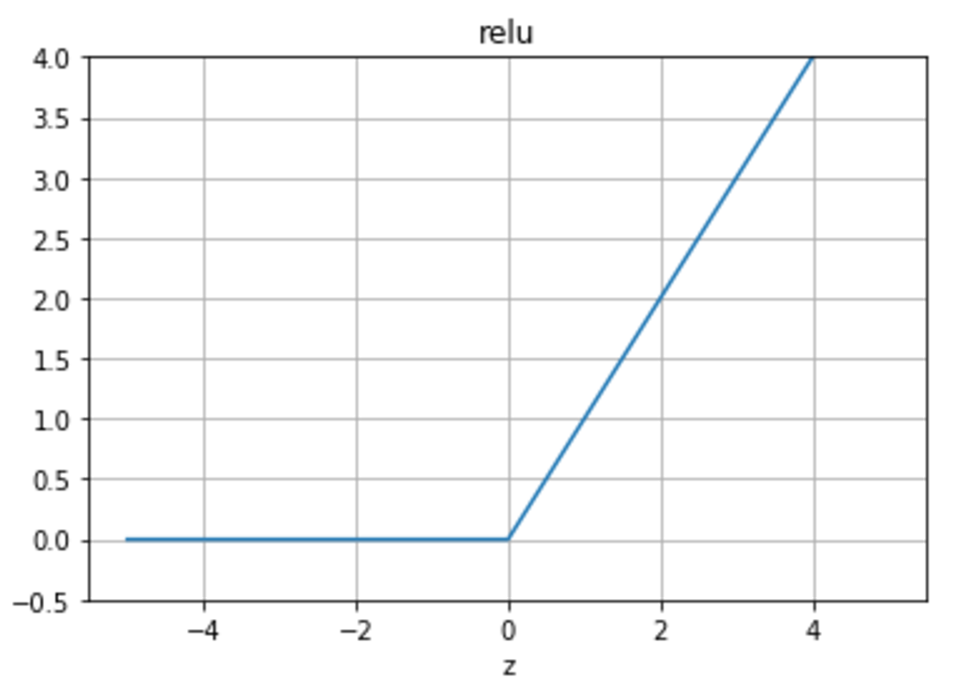Thanks to the properties of its derivative, which is, there are two main advantages of using the ReLU activation function over sigmoid:

• Faster learning because of constant value of relu'(z), compared to that of the logistic function.
• Less likely to have the vanishing gradient problem, exponential decrease of gradient, which can be found in networks with multiple stacked sigmoid layers. As we multiply the derivative of the activation function when calculating errors δ for each layer, and the maximal value of sigmoid'(z) is ¼, the gradients will decrease exponentially as we stack more and more sigmoid layers.

The `nnet` package we used in previous sections is (by now) only capable of modeling a single-layer network. In this chapter, we use the `MXNet` package to implement deep neural networks with multiple hidden layers. `MXNet` (https://mxnet.incubator.apache.org/) is a deep learning framework that supports programming languages include R, Scala, Python, Julia, C++, and Perl. It is developed by the DMLC (http://dmlc.ml/) team, a group of experts collaborating on open-source machine learning projects. It is portable and can scale to multiple CPUs, multiple GPUs and multiple machines, for example, in the cloud. Most importantly, it allows us to flexibly and efficiently construct state-of-the-art deep learning models, including deep neural networks, CNNs and RNNs.

Let's install `MXNet` first:

```> cran <- getOption("repos")
> cran["dmlc"] <- "https://s3-us-west-2.amazonaws.com/apache-mxnet/R/CRAN/"
> options(repos = cran)
> if (!require("mxnet"))
install.packages("mxnet")```

Now we can import `MXNet` and convert the data into the format preferred by the neural network models in `MXNet`:

```> require(mxnet)
> data_train <- data.matrix(data_train)
> data_train.x <- data_train[,-1]
> data_train.x <- t(data_train.x/255)
> data_train.y <- data_train[,1]```

Note we scale the input features to a range from `0` to `1`, by dividing the maximal possible value `255`. Otherwise, the deep neural networks may be skewed towards some features and such skewness will accumulate over layers.

Now that the training dataset is ready, we can start constructing the network by defining its architecture as follows:

```> data <- mx.symbol.Variable("data")
> fc1 <- mx.symbol.FullyConnected(data, name="fc1", num_hidden=128)
> act1 <- mx.symbol.Activation(fc1, name="relu1", act_type="relu")
> fc2 <- mx.symbol.FullyConnected(act1, name="fc2", num_hidden=64)
> act2 <- mx.symbol.Activation(fc2, name="relu2", act_type="relu")
> fc3 <- mx.symbol.FullyConnected(act2, name="fc3", num_hidden=10)
> softmax <- mx.symbol.SoftmaxOutput(fc3, name="sm")```

In the MXNet's Symbol API, we represent the network in the data type symbol. We begin with the input layer data, the input data, and follow up with the first hidden layer `fc1` with 128 nodes, which fully connects with the input layer. We then attach the ReLU function to `fc1` and output the activations `act1` for this layer. Similarly, we chain another hidden layer `fc2`, with 64 nodes this time, and output ReLU-based activates `act2`. Finally, we end up with the output layer with a softmax function, generating 10 probabilities corresponding to 10 classes. The overall structure looks like this: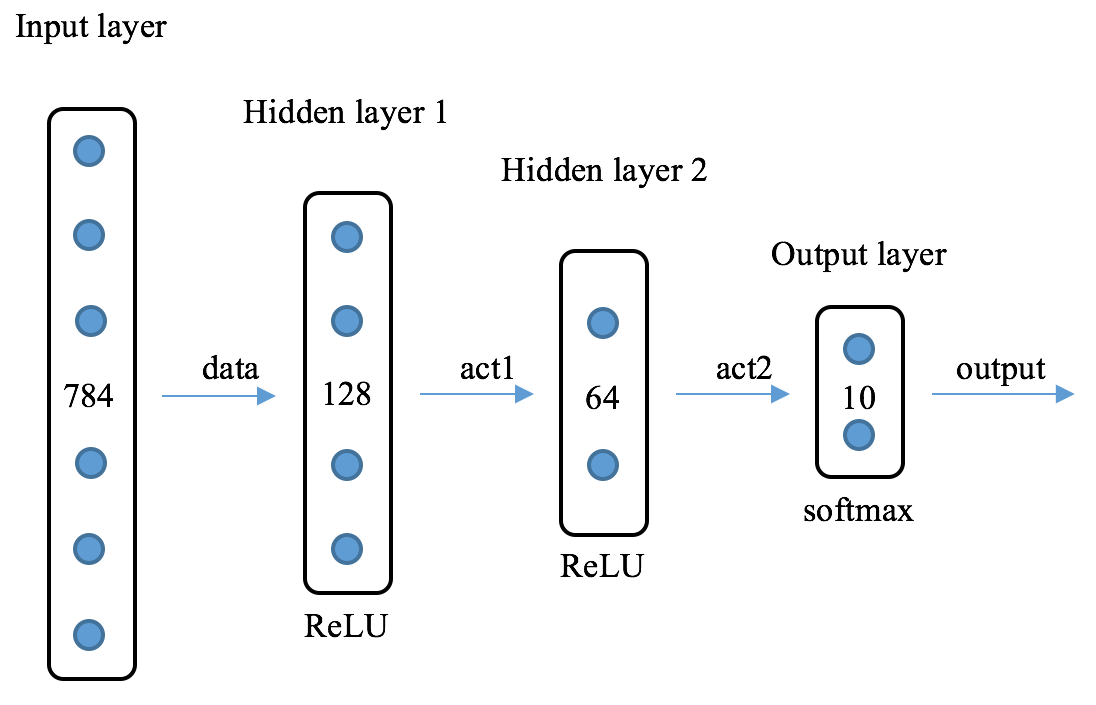After building the bone, it is time to train the model. We can choose our computation device, CPU and/or GPU—here is a CPU example:

`> devices <- mx.cpu()`

Before training, don't forget to set the random seed to make the modeling process reproducible:

```> mx.set.seed(42)
> model_dnn <- mx.model.FeedForward.create(softmax, X=data_train.x,
y=data_train.y, ctx=devices, num.round=30, array.batch.size=100,
learning.rate=0.01, momentum=0.9, eval.metric=mx.metric.accuracy,
initializer=mx.init.uniform(0.1),
epoch.end.callback=mx.callback.log.train.metric(100))
Start training with 1 devices
 Train-accuracy=0.724793650793651
 Train-accuracy=0.904715189873417
 Train-accuracy=0.925537974683544
 Train-accuracy=0.939936708860759
 Train-accuracy=0.950379746835443
 Train-accuracy=0.95873417721519
 Train-accuracy=0.96509493670886
 Train-accuracy=0.969905063291139
 Train-accuracy=0.974303797468355
 Train-accuracy=0.977784810126584
 Train-accuracy=0.980696202531648
 Train-accuracy=0.983164556962027
 Train-accuracy=0.985284810126584
 Train-accuracy=0.987405063291141
 Train-accuracy=0.988924050632913
 Train-accuracy=0.990727848101267
 Train-accuracy=0.992088607594938
 Train-accuracy=0.993227848101268
 Train-accuracy=0.994398734177217
 Train-accuracy=0.995284810126584
 Train-accuracy=0.995854430379748
 Train-accuracy=0.996835443037975
 Train-accuracy=0.997183544303798
 Train-accuracy=0.997848101265823
 Train-accuracy=0.998164556962026
 Train-accuracy=0.998575949367089
 Train-accuracy=0.998924050632912
 Train-accuracy=0.999177215189874
 Train-accuracy=0.999367088607595
 Train-accuracy=0.999525316455696```

We just fit the model with hyperparameters including:

• `num.round = 30`: The maximum number of iterations is set to be `30`.
• `array.batch.size = 100`: The batch size of the mini-batch gradient descent is 100. As a variation of a stochastic gradient descent, the mini-batch gradient descent algorithm calculates costs and gradients by small batches, instead of individual training samples. Hence, it is computationally more efficient and allows faster model convergence. As a result, the mini-batch gradient descent is more commonly used in training deep neural networks.
• `learning.rate = 0.01`: The learning rate is `0.01`.
• `momentum=0.9`: In general, the cost function of deep architectures has the form of one or more shallow ravines (local minima) leading to the global optimum. Momentum as seen in the physical law of motion is employed to avoid getting stuck in sub-optimum and make the convergence faster. With momentum, weights are updated as follows:where the left and right v is the previous and current velocity respectively, and γ ∈ (0,1] is the momentum factor determining how much of the previous velocity is incorporated into the current one.

• `eval.metric=mx.metric.accuracy`: It uses classification accuracy as the evaluation metric
• `initializer=mx.init.uniform(0.1)`: Initial weights are randomly generated from the uniform distribution ranging from 0 to 1, so as to lower the chances of the weight exploding and vanishing in the deep network

After the model is trained, let's see how it performs on the testing set. First, remember to conduct the same pre-processing on the test dataset:

```> data_test.x <- data_test[,-1]
> data_test.x <- t(data_test.x/255)```

Then, predict the testing cases and evaluate the performance:

```> prob_dnn <- predict(model_dnn, data_test.x)
> prediction_dnn <- max.col(t(prob_dnn)) - 1
> cm_dnn = table(data_test\$label, prediction_dnn)```
```> cm_dnn
prediction_dnn
0    1    2    3    4    5    6    7    8    9
0 1041    0    2    0    0    1    3    0    8    1
1    0 1157    3    1    1    0    1    3    1    0
2    2    1  993    3    3    1    2   13    5    2
3    1    3   14 1033    1   13    0    5   14    6
4    0    2    1    0  991    0    4    4    1   12
5    4    2    3   12    3  892    4    3    6    8
6   10    0    1    0    3    4  988    0    4    0
7    0    5    9    1    2    0    0 1116    2    1
8    4    8    3    5    0    8    3    2 1020   12
9    1    1    0    4   13    3    0   16    2  957
> accuracy_dnn = mean(prediction_dnn == data_test\$label)
> accuracy_dnn
 0.9704706 ```

By adding one more hidden layer, accuracy is improved from 91.4% to 97.0%! Since each hidden layer in a deep neural network provides representations of the data at a certain level, can we simply conclude that the more hidden layers (such as 100, 1,000, 10,000...), the more underneath patterns are discovered, the better the classification accuracy? It might be true if we have plentiful resources and time to enable computation and to make sure overfitting does not occur with such complex networks. Is there any way where we can extract richer and more informative representations than by simply chaining more hidden layers, and at the same time, not excessively grow our networks? The answer is CNNs.

### Extracting richer representation with CNNs

Although regular hidden layers (we also call them fully connected layers) do the job of obtaining representations at certain levels, these representations might be able to help us differentiate between images of different classes. We need to extract richer and distinguishable representations that, for example, make a "9" a "9", a "4" a "4", or a cat a cat, a dog a dog. We resort to CNNs as variants of multi-layered neural networks which are biologically inspired by the human visual cortex. Basically, CNNs take inspiration from the following two neuroscience findings:

• The visual cortex has a complex system of neuronal cells that are sensitive to specific sub-regions of the visual field, called the receptive field. For instance, some cells respond only in the presence of vertical edges; some cells fire only when exposed to horizontal edges; and some react more strongly when shown edges of a certain orientation. The cells are organized together to produce the entire visual perception, while each individual cell is specialized in a specific component.
• Simple cells respond only when those edge-like patterns are presented within their receptive sub-regions. More complex cells are sensitive to larger sub-regions, and as a result, are less variant to the local position of those edge-like patterns in the entire visual field.

Similarly, CNNs classify images by first deriving low-level representations, local edges and curves, then by composing higher-level representations, overall shape and contour, through a series of low-level representations. CNNs are well suited to exploiting strong and unique features that differentiate between images.

In general, CNNs take in an image, pass it through a sequence of convolutional layers, non-linear layers, pooling layers and fully connected layers, and finally output the probabilities of each possible class. We now look at each type of layer individually, in detail.

The convolutional layer is the first layer in a CNN. It simulates the way neuronal cells respond to receptive fields by applying a convolutional operation to the input. To be specific, it computes the dot product between the weights of the convolutional layer and a small region they are connected to in the input layer. The small region is the receptive field, and the weights can be viewed as the values on a filter. As the filter slides from the beginning to the end of the input layer, the dot product between the weights and current receptive field is computed. A new layer called feature map is obtained after convolving over all sub-regions. Take a look at the following example: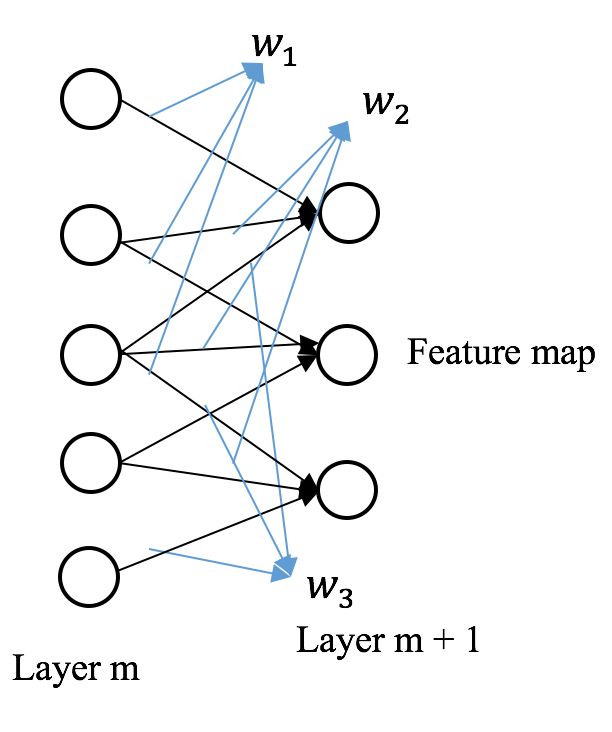Layer m has five nodes and the filter has three units [w1, w2, w3]. We compute the dot product between the filter and the first three nodes in layer m and obtain the first node in the feature map; then, we compute the dot product between the filter and the middle three nodes and generate the second node; finally, we obtain the third node resulting from the last three nodes in layer m.

Another example that helps us better understand how the convolutional layer works on images is as follows: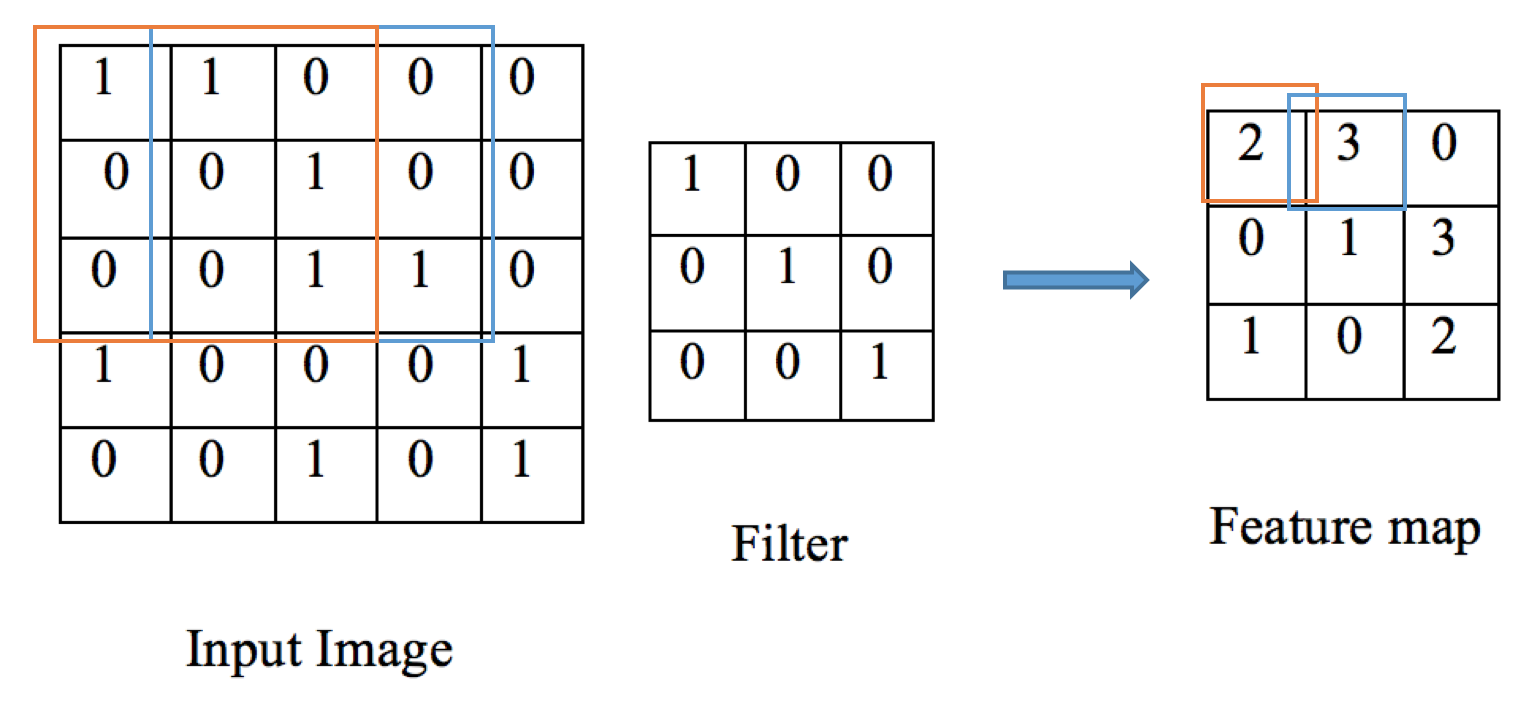A 3*3 filter is sliding around a 5*5 image from the top left sub-region to the bottom right. For every sub-region, the dot product is computed with the filter. A 3*3 feature map is generated as a result.

Convolutional layers are actually used to extract features, such as edges and curves. The output pixel in the feature map will be of high value, if the corresponding receptive field contains an edge or curve specified by the filter. For instance, in the preceding example, the filter portrays a backslash-shape diagonal edge, the receptive field in the blue rectangle contains a similar curve and hence, the highest intensity 3 (1*1 + 1* 1 + 1*1 = 3) is produced; however, the receptive field in the bottom left corner does not contain such a shape, which results in a pixel of value 1. The convolutional layer acts as a curve detector, mimicking the way our visual cells work.

Remember in the preceding case, we only applied one filter and generated one feature map, which indicates how well the shape in the input image resembles the curve represented in the filter. To achieve a richer representation of the data, we can employ more filters, such as horizontal, vertical curve, 30-degree, or right-angle shape, so that the hidden layer composed of feature maps can detect more patterns. Additionally, stacking many convolutional layers can produce higher-level representations, such as overall shape and contour. To ensure the strong spatially local patterns are caught, each filter in a layer is only responsive to the corresponding receptive fields. Chaining more layers results in larger receptive fields which capture more global patterns.

Right after each convolutional layer, we often apply a non-linear layer (also called activation layer, as we mentioned), in order to introduce non-linearity, obviously. This is because only linear operations (multiplication and addition) are conducted in the convolutional layer. And a neural network with only linear hidden layers would behave just like a single-layer perceptron, regardless of how many layers. Again, ReLu is the most popular candidate for the non-linear layer in deep neural networks.

Normally, after obtaining features via one or more pairs of convolutional layers and non-linear layers, we can use the output for classification, for example applying a softmax layer in our multiclass case. Let's do some math, suppose we apply 20 5*5 filters in the first convolutional layer, then the output of this layer will be of size 20 * (28 - 5 + 1) * (28 - 5 + 1) = 20 * 24 * 24 = 11520, which means the number of features as inputs for the next layer becomes 11,520 from 784; we then apply 50 5*5 filters in the second convolutional layer, the size of the output grows to 50 * 20 * (24 - 5 + 1) * (24 - 5 + 1) = 400,000, which is high-dimensional compared to our initial size of 784. We can see that the dimension increases dramatically with each convolutional layer before the softmax layer for classification. This can easily lead to overfitting, not to mention the large number of weights to be trained in the corresponding non-linear layer.

To address the dimension growth issue, we often employ a pooling layer (also called downsampling layer) after a pair of convolutional and non-linear layers. As its name implies, it aggregates statistics of features by sub-regions to generate much lower dimensional features. Typical pooling methods include max pooling and mean pooling, which take the max values and mean values over all non-overlapping sub-regions, respectively. For example, we apply a 2*2 max pooling filter on a 4*4 feature map and output a 2*2 one: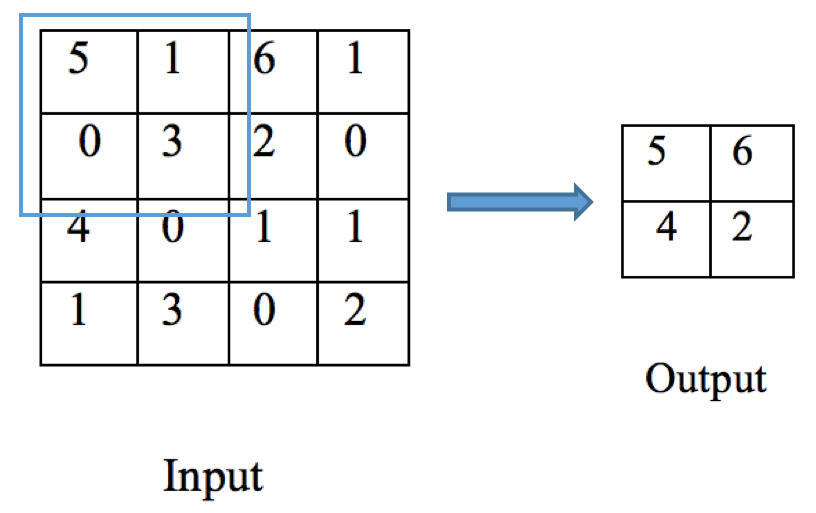Besides reducing overfitting with lower dimensional output, the pooling layer aggregating statistics over regions has another advantage—translation invariant. It means the output does not change, if the input image undergoes a small amount of translation. For example, suppose we shift the input image to a couple of pixels left or right, up or down, as long as the highest pixels remain the same in sub-regions, the output of the max pooling layer is still the same. In another words, the prediction becomes less position-sensitive with pooling layers.

Putting these three types of convolutional-related layers together, along with fully connected layers, we can structure our CNN model as follows: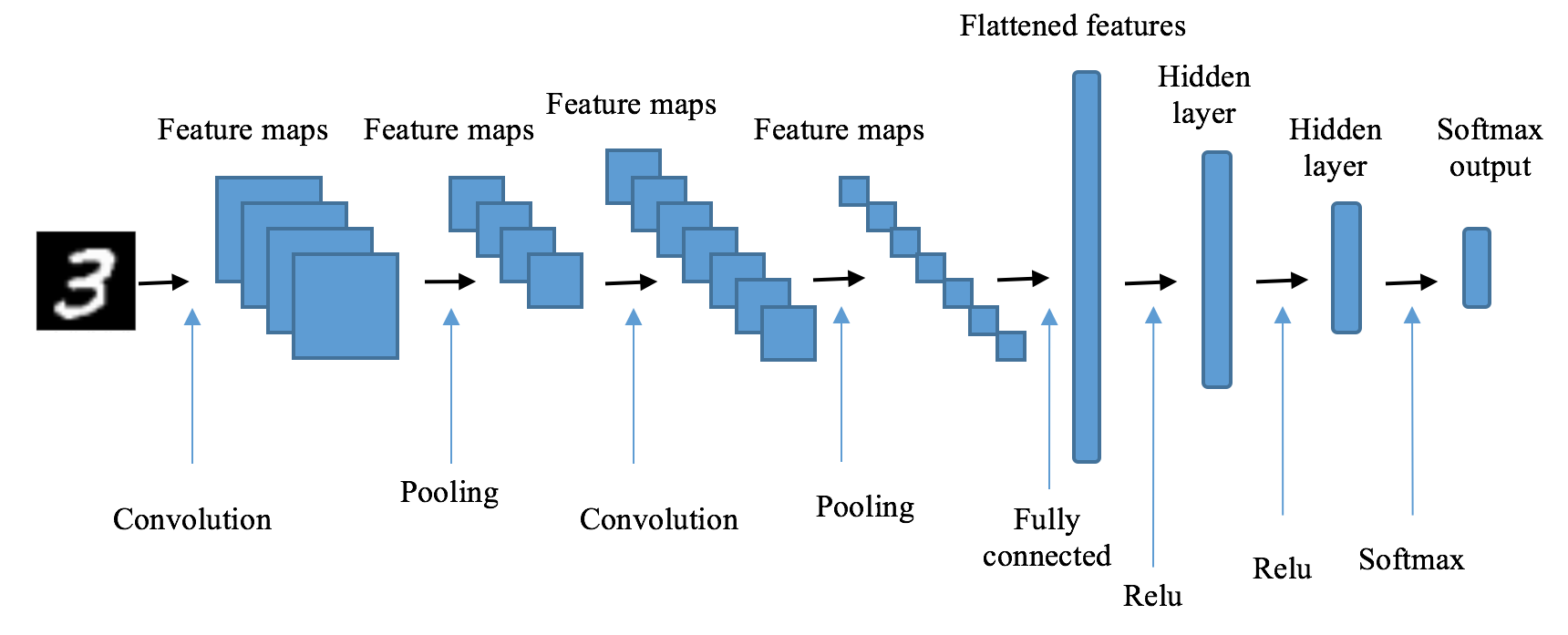It starts with the first convolutional layer, ReLu non-linear layer and pooling layer. Here, we use 20 5*5 convolutional filters, and a 2*2 max pooling filter:

```> # first convolution
> conv1 <- mx.symbol.Convolution(data=data, kernel=c(5,5),
num_filter=20)
> act1 <- mx.symbol.Activation(data=conv1, act_type="relu")
> pool1 <- mx.symbol.Pooling(data=act1, pool_type="max",
+ kernel=c(2,2), stride=c(2,2))```

It follows with the second convolutional, ReLu non-linear and pooling layer, where 50 5*5 convolutional filters and a 2*2 max pooling filter are used:

```> # second convolution
> conv2 <- mx.symbol.Convolution(data=pool1, kernel=c(5,5),
num_filter=50)
> act2 <- mx.symbol.Activation(data=conv2, act_type="relu")
> pool2 <- mx.symbol.Pooling(data=act2, pool_type="max",
+ kernel=c(2,2), stride=c(2,2))```

Now that we extract rich representations of the input images by detecting edges, curves and shapes, we move on with the fully connected layers. But before doing so, we need to flatten the resulting feature maps from previous convolution layers:

`> flatten <- mx.symbol.Flatten(data=pool2)`

In the fully connected section, we apply two ReLu hidden layers with 500 and 10 units respectively:

```> # first fully connected layer
> fc1 <- mx.symbol.FullyConnected(data=flatten, num_hidden=500)
> act3 <- mx.symbol.Activation(data=fc1, act_type="relu")
> # second fully connected layer
> fc2 <- mx.symbol.FullyConnected(data=act3, num_hidden=10)```

Finally, the softmax layer producing outputs for 10 classes:

```> # softmax output
> softmax <- mx.symbol.SoftmaxOutput(data=fc2, name="sm")```

Now, the bone is constructed. Time to set a random seed and start training the model:

We need to first reshape the matrix, `data_train.x` into an array as required by the convolutional layer in `MXNet`:

```> train.array <- data_train.x
> dim(train.array) <- c(28, 28, 1, ncol(data_train.x))
> mx.set.seed(42)
> model_cnn <- mx.model.FeedForward.create(softmax, X=train.array,
y=data_train.y, ctx=devices, num.round=30,
array.batch.size=100, learning.rate=0.05,
momentum=0.9, wd=0.00001,
eval.metric=mx.metric.accuracy,
epoch.end.callback=mx.callback.log.train.metric(100))
Start training with 1 devices
 Train-accuracy=0.306984126984127
 Train-accuracy=0.961898734177216
 Train-accuracy=0.981139240506331
 Train-accuracy=0.987151898734179
 Train-accuracy=0.990348101265825
 Train-accuracy=0.992689873417723
 Train-accuracy=0.994493670886077
 Train-accuracy=0.995822784810128
 Train-accuracy=0.995601265822786
 Train-accuracy=0.997246835443039
 Train-accuracy=0.997341772151899
 Train-accuracy=0.998006329113925
 Train-accuracy=0.997626582278482
 Train-accuracy=0.998069620253165
 Train-accuracy=0.998765822784811
 Train-accuracy=0.998449367088608
 Train-accuracy=0.998765822784811
 Train-accuracy=0.998955696202532
 Train-accuracy=0.999746835443038
 Train-accuracy=0.999841772151899
 Train-accuracy=0.999905063291139
 Train-accuracy=1
 Train-accuracy=1
 Train-accuracy=1
 Train-accuracy=1
 Train-accuracy=1
 Train-accuracy=1
 Train-accuracy=1
 Train-accuracy=1
 Train-accuracy=1```

Besides those hyperparameters we used in the previous deep neural network model, we fit the CNN model with L2 regularization weight decay `wd = 0.00001`, which adds penalties for large weights in order to avoid overfitting.

Again, training of the CNN model is no different to other networks. Optimal weights are obtained through a backpropagation algorithm.

After the model is trained, let's see how it performs on the testing set. First, remember to conduct the same pre-processing on the test dataset:

```> test.array <- data_test.x
> dim(test.array) <- c(28, 28, 1, ncol(data_test.x))```

Predict the testing cases and evaluate the performance:

```> prob_cnn <- predict(model_cnn, test.array)
> prediction_cnn <- max.col(t(prob_cnn)) - 1
> cm_cnn = table(data_test\$label, prediction_cnn)
> cm_cnn
prediction_cnn
0 1 2 3 4 5 6 7 8 9
0 1051 0 1 0 0 1 1 0 2 0
1 0 1161 0 0 0 1 1 3 1 0
2 0 0 1014 4 0 0 0 7 0 0
3 0 0 2 1075 0 6 0 2 3 2
4 0 0 0 0 1000 0 4 2 2 7
5 1 0 0 4 0 923 3 0 3 3
6 3 0 0 0 0 0 1006 0 1 0
7 0 1 2 0 3 0 0 1129 1 0
8 3 3 1 1 2 5 1 0 1043 6
9 2 0 2 0 3 3 1 2 0 984
> accuracy_cnn = mean(prediction_cnn == data_test\$label)
> accuracy_cnn
 0.9893313```

Our CNN model further boosts the accuracy to close to 99%!

We can also view the network structure by:

`> graph.viz(model_cnn\$symbol)`

Let's do some more inspection to make sure we get things right. We start with the learning curving, for example the classification performance of the model on both the training set and testing set over the number of training iterations. In general, it is a good practice to plot the learning curve where we can visualize whether overfitting or underfitting issues occur:

```> data_test.y <- data_test[,1]
> logger <- mx.metric.logger\$new()
> model_cnn <- mx.model.FeedForward.create(softmax, X=train.array,
y=data_train.y,eval.data=list(data=test.array,
label=data_test.y), ctx=devices, num.round=30,
array.batch.size=100, learning.rate=0.05,
momentum=0.9, wd=0.00001,eval.metric=
mx.metric.accuracy, epoch.end.callback =
mx.callback.log.train.metric(1, logger))
Start training with 1 devices
 Train-accuracy=0.279936507936508
 Validation-accuracy=0.912857142857143
 Train-accuracy=0.959462025316456
 Validation-accuracy=0.973523809523809
 Train-accuracy=0.979841772151899
 Validation-accuracy=0.980666666666666
 Train-accuracy=0.986677215189875
 Validation-accuracy=0.983428571428571
 Train-accuracy=0.990822784810129
 Validation-accuracy=0.981809523809523
 Train-accuracy=0.992626582278482
 Validation-accuracy=0.983904761904761
 Train-accuracy=0.993322784810128
 Validation-accuracy=0.986
 Train-accuracy=0.995474683544305
 Validation-accuracy=0.987619047619047
 Train-accuracy=0.996487341772153
 Validation-accuracy=0.983904761904762
 Train-accuracy=0.995949367088608
 Validation-accuracy=0.984761904761904
 Train-accuracy=0.997310126582279
 Validation-accuracy=0.985142857142856
 Train-accuracy=0.997658227848102
 Validation-accuracy=0.986857142857142
 Train-accuracy=0.997848101265824
 Validation-accuracy=0.984095238095238
 Train-accuracy=0.998006329113924
 Validation-accuracy=0.985238095238094
 Train-accuracy=0.998607594936709
 Validation-accuracy=0.987619047619047
 Train-accuracy=0.99863924050633
 Validation-accuracy=0.987428571428571
 Train-accuracy=0.998987341772152
 Validation-accuracy=0.985142857142857
 Train-accuracy=0.998765822784811
 Validation-accuracy=0.986285714285713
 Train-accuracy=0.999240506329114
 Validation-accuracy=0.988761904761905
 Train-accuracy=0.999335443037975
 Validation-accuracy=0.98847619047619
 Train-accuracy=0.999841772151899
 Validation-accuracy=0.987809523809523
 Train-accuracy=0.99993670886076
 Validation-accuracy=0.990095238095237
 Train-accuracy=1
 Validation-accuracy=0.989999999999999
 Train-accuracy=1
 Validation-accuracy=0.989999999999999
 Train-accuracy=1
 Validation-accuracy=0.990190476190476
 Train-accuracy=1
 Validation-accuracy=0.990190476190476
 Train-accuracy=1
 Validation-accuracy=0.990095238095237
 Train-accuracy=1
 Validation-accuracy=0.990095238095237
 Train-accuracy=1
 Validation-accuracy=0.990095238095237
 Train-accuracy=1
 Validation-accuracy=0.990190476190475```

We can get the performance on the training set after each round of training:

```> logger\$train
 0.2799365 0.9594620 0.9798418 0.9866772 0.9908228 0.9926266 0.9933228 0.9954747 0.9964873 0.9959494 0.9973101
 0.9976582 0.9978481 0.9980063 0.9986076 0.9986392 0.9989873 0.9987658 0.9992405 0.9993354 0.9998418 0.9999367
 1.0000000 1.0000000 1.0000000 1.0000000 1.0000000 1.0000000 1.0000000 1.0000000```

As well as the performance on the testing set after each round of training:

```> logger\$eval
 0.9128571 0.9735238 0.9806667 0.9834286 0.9818095 0.9839048 0.9860000 0.9876190 0.9839048 0.9847619 0.9851429
 0.9868571 0.9840952 0.9852381 0.9876190 0.9874286 0.9851429 0.9862857 0.9887619 0.9884762 0.9878095 0.9900952
 0.9900000 0.9900000 0.9901905 0.9901905 0.9900952 0.9900952 0.9900952 0.9901905```

The learning curve can be visualized by the following codes:

```> plot(logger\$train,type="l",col="red", ann=FALSE)
> lines(logger\$eval,type="l", col="blue")
> title(main="Learning curve")
> title(xlab="Iterations")
> title(ylab="Accuary")
> legend(20, 0.5, c("training","testing"), cex=0.8,
col=c("red","blue"), pch=21:22, lty=1:2);```

And we will get: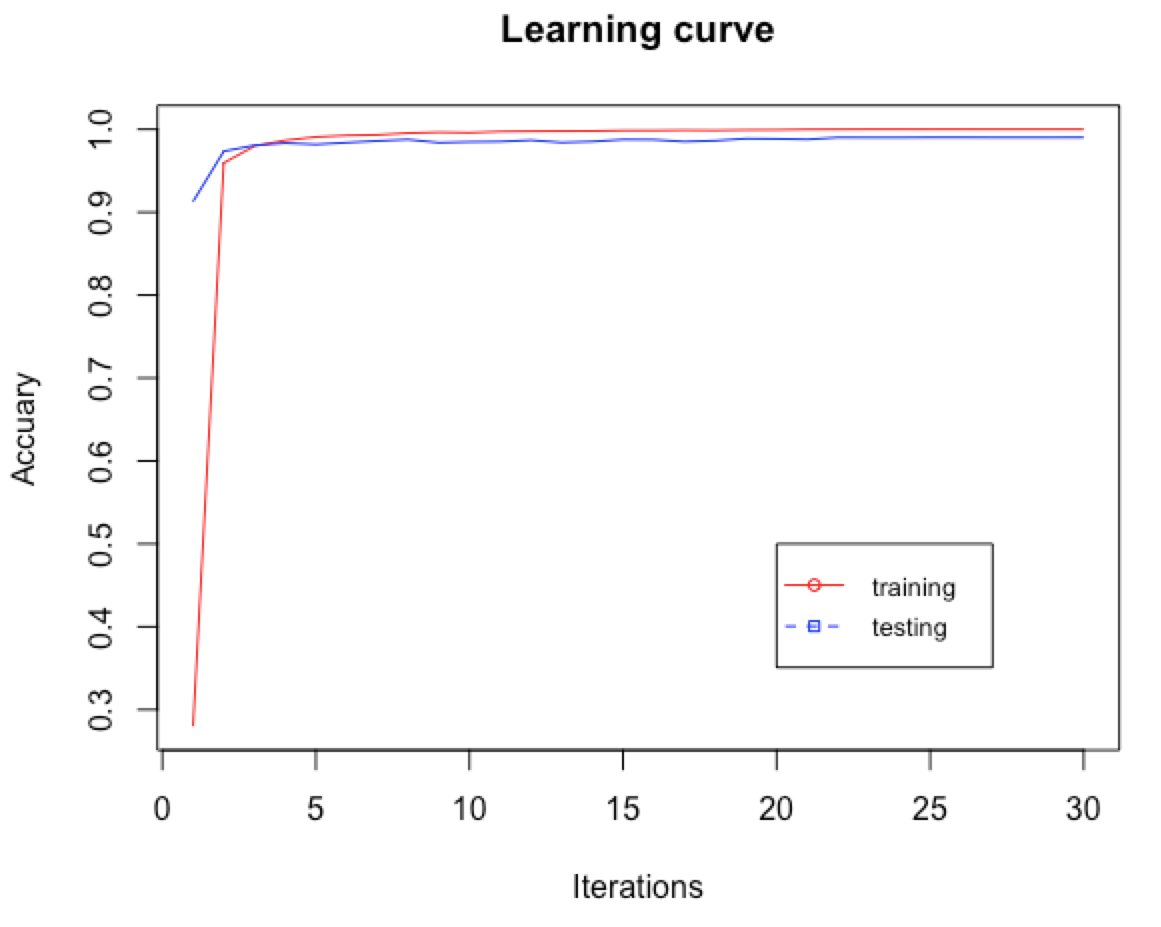The learning curve indicates little chance of overfitting or underfitting.

Since the model works great, why don't we visualize the output of the convolutional layers of the trained model so that we can get a better understanding of CNNs. Let's use the first two samples in the testing set as an example. They are `1` and `0`:

```> par(mfrow=c(1,2))
> test_1 <- matrix(as.numeric(data_test[1,-1]), nrow = 28,
byrow = TRUE)
> image(rotate(test_1), col = grey.colors(255))
> test_2 <- matrix(as.numeric(data_test[2,-1]), nrow = 28,
byrow = TRUE)
> image(rotate(test_2), col = grey.colors(255))```

For our reference, they are displayed as: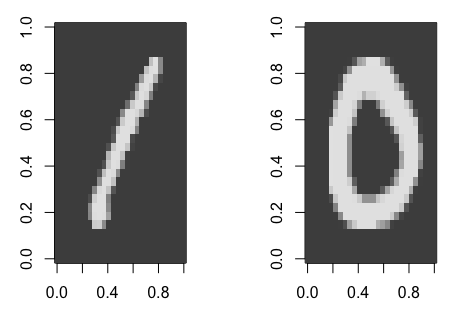To visualize the activation of the convolutional layers, we first create an executor (can be loosely viewed as a copy of our trained CNN model) by grouping all of the layers with activations:

```> layerss_for_viz <- mx.symbol.Group(mx.symbol.Group(c(conv1, act1, pool1, conv2, act2, pool2, fc1, fc2)))
data=dim(test.array), ctx=mx.cpu())```

Now, update the weights in the executor with those in the trained model:

```> mx.exec.update.arg.arrays(executor, model_cnn\$arg.params, match.name=TRUE)
> mx.exec.update.aux.arrays(executor, model_cnn\$aux.params,
match.name=TRUE)```

And apply the executor on the testing set by making a feed-forward pass:

```> mx.exec.update.arg.arrays(executor,
list(data=mx.nd.array(test.array)), match.name=TRUE)
> mx.exec.forward(executor, is.train=FALSE)```

We can see the names of the layers recorded in the executor, as we will be extracting the activation of a layer by its name (note the names can be different, we should use the corresponding ones):

```> names(executor\$ref.outputs)
 "convolution10_output" "activation15_output"
"pooling10_output" "convolution11_output"
 "activation16_output" "pooling11_output"
"fullyconnected10_output" "fullyconnected11_output"```

Now, we can visualize the activations for the first and second convolutional layer and ReLu layer, as well as the first pooling layer.

Let's start with the ReLu activations for the first 16 filters in the first convolutional layer, which are called `activation15_output` in our case (again, the name may vary). For the first sample, `(a "1")`, we run the following scripts:

```> par(mfrow=c(4,4), mar=c(0.1,0.1,0.1,0.1))
> for (i in 1:16) {
+ outputData <- as.array
(executor\$ref.outputs\$activation15_output)[,,i,1]
+ image(outputData, xaxt='n', yaxt='n',
col=grey.colors(255)
+ )
+ }```

Similarly, for the second sample, `(a "0")`, we run:

```> par(mfrow=c(4,4), mar=c(0.1,0.1,0.1,0.1))
> for (i in 1:16) {
+ outputData <- as.array
(executor\$ref.outputs\$activation15_output)[,,i,2]
+ image(outputData, xaxt='n', yaxt='n',
col=grey.colors(255)
+ )
+ }```

We plot the activations of the first convolutional layer for a 1 (left) and a 0 (right) input image, respectively: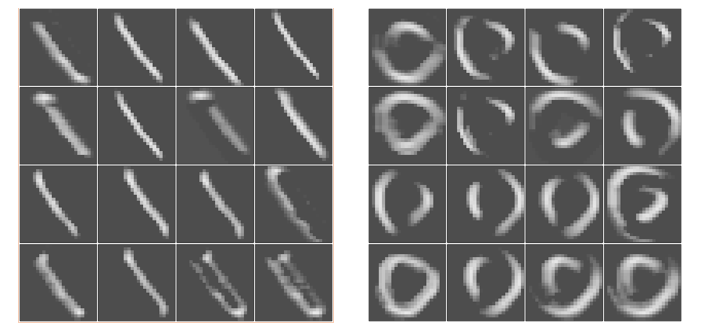We can observe that each feature map effectively extracts the edges, curves and strikes of the digits.

We continue with the corresponding outputs of the first pooling layer called `pooling10_output`:

```> par(mfrow=c(4,4), mar=c(0.1,0.1,0.1,0.1))
> for (i in 1:16) {
+ outputData <-as.array
(executor\$ref.outputs\$pooling10_output)[,,i,1]
+ image(outputData, xaxt='n', yaxt='n',
col=grey.colors(255)
+ )
+ }
> par(mfrow=c(4,4), mar=c(0.1,0.1,0.1,0.1))
> for (i in 1:16) {
+ outputData <- as.array
(executor\$ref.outputs\$pooling10_output)[,,i,2]
+ image(outputData, xaxt='n', yaxt='n',
col=grey.colors(255)
+ )
+ }```

We plot the outputs of the first max pooling layer: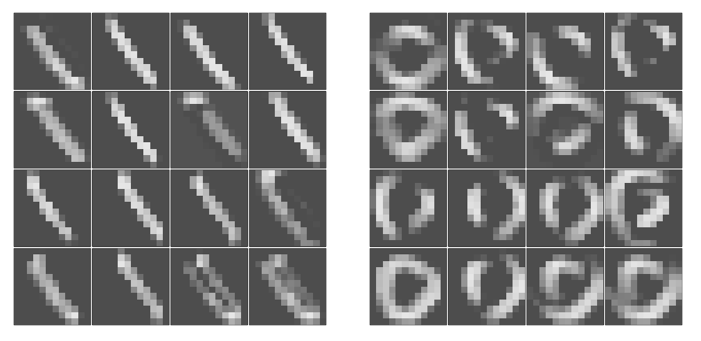As we can easily tell, they are the downsampled versions of the convolution outputs.

Finally, we visualize one more layer, the second convolutional layer, which is labeled as `convolution11_output`. Take the first 16 feature maps as an example:

```> par(mfrow=c(4,4), mar=c(0.1,0.1,0.1,0.1))
> for (i in 1:16) {
+ outputData <- as.array
(executor\$ref.outputs\$convolution11_output)[,,i,1]
+ image(outputData, xaxt='n', yaxt='n',
col=grey.colors(255)
+ )
+ }
> par(mfrow=c(4,4), mar=c(0.1,0.1,0.1,0.1))
> for (i in 1:16) {
+ outputData <- as.array
(executor\$ref.outputs\$convolution11_output)[,,i,2]
+ image(outputData, xaxt='n', yaxt='n',
col=grey.colors(255)
+ )
+ }```

We plot the outputs of the second convolutional layer for two images, respectively: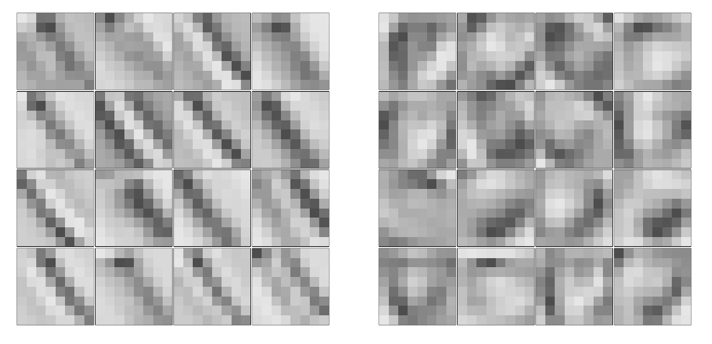The second convolutional layer extracts higher-level features of the input images, such as shape and contour. All these 50 feature maps combined together, provide rich representations which are then fed into the fully connected layers. Therefore, classification performance is increased thanks to the convolution operations.

So far, we have examined the effectiveness of our CNN model by plotting the learning curve and visualizing the convolution extracted features. We can do one more inspection on the generalization of our model. For instance, we can take out a subset of data from the testing set to simulate a validation set, and use the remainder as the new testing set. We perform early stopping based on the validation set, which provides instruction on how many iterations is good enough and does not overfit the model. Finally, we employ the trained model with early stopping on the testing set and see how well the model is able to generalize to unseen data.

We first split the testing set into validation (40%) and final testing set (60%):

```> validation_perc = 0.4
> validation_index <- createDataPartition(data_test.y, p=validation_perc, list=FALSE)
>
> validation.array <- test.array[, , , validation_index]
> dim(validation.array) <- c(28, 28, 1,
length(validation.array[1,1,]))
> data_validation.y <- data_test.y[validation_index]
> final_test.array <- test.array[, , , -validation_index]
> dim(final_test.array) <- c(28, 28, 1,
length(final_test.array[1,1,]))
> data_final_test.y <- data_test.y[-validation_index]```

To conduct early stopping, we write our custom callback function and will assign it to the parameter, `epoch.end.callback`. The callback function checks the classification performance on the validation set, and if it is greater than the threshold we set, the training stops:

```> mx.callback.early.stop <- function(eval.metric) {
+ function(iteration, nbatch, env, verbose) {
+ if (!is.null(env\$metric)) {
+ if (!is.null(eval.metric)) {
+ result <- env\$metric\$get(env\$eval.metric)
+ if (result\$value >= eval.metric) {
+ return(FALSE)
+ }
+ }
+ }
+ return(TRUE)
+ }
+ }```

Now, we train the CNN model with early stopping based on the validation set where we set the stopping criteria as a validation accuracy greater than `0.985`:

```> model_cnn_earlystop <- mx.model.FeedForward.create(softmax,
X=train.array, y=data_train.y,
eval.data=list(data=validation.array, label=data_validation.y),
+ ctx=devices, num.round=30, array.batch.size=100,
+ learning.rate=0.05, momentum=0.9, wd=0.00001,
eval.metric=mx.metric.accuracy,
+ epoch.end.callback = mx.callback.early.stop(0.985))
Start training with 1 devices
 Train-accuracy=0.284571428571429
 Validation-accuracy=0.921395348837209
 Train-accuracy=0.959145569620254
 Validation-accuracy=0.972325581395349
 Train-accuracy=0.980221518987343
 Validation-accuracy=0.97906976744186
 Train-accuracy=0.986613924050634
 Validation-accuracy=0.982790697674419
 Train-accuracy=0.990537974683546
 Validation-accuracy=0.981627906976744
 Train-accuracy=0.992848101265824
 Validation-accuracy=0.985348837209302```

Training stops after the sixth iteration as the criteria is met. Finally, the performance on the new testing set is examined:

```> prob_cnn <- predict(model_cnn_earlystop, final_test.array)
> prediction_cnn <- max.col(t(prob_cnn)) - 1
> cm_cnn = table(data_final_test.y, prediction_cnn)
> cm_cnn
prediction_cnn
data_final_test.y 0 1 2 3 4 5 6 7 8 9
0 626 0 0 0 0 0 0 0 1 0
1 0 701 1 0 0 2 1 3 4 0
2 1 0 598 4 0 0 0 6 0 0
3 0 0 0 658 0 5 0 0 2 1
4 0 0 0 0 585 1 3 3 1 4
5 1 0 1 3 0 558 5 0 0 2
6 4 0 0 0 0 0 595 0 0 0
7 0 1 3 0 1 0 0 675 0 0
8 4 0 1 1 1 7 3 0 621 2
9 1 0 0 0 2 1 0 4 0 589
> accuracy_cnn = mean(prediction_cnn == data_final_test.y)
> accuracy_cnn
 0.9855487```

Our CNN model is able to generalize decently.

## Summary

We have just finished our first mile in the R and deep learning journey! Through this chapter, we got more familiar with the important concepts of deep learning. We started with what deep learning is all about, why it is important and the recent success of applications, as well. After we were well equipped, we solved the handwritten digit using shallow neural networks, deep neural networks and CNNs in sequence, and proved that CNNs are the best suited to exploiting strong and unique features that differentiate images of different classes.

Inspired by the human visual cortex, CNNs classify images by first deriving rich representations such as edges, curves and shapes, which was demonstrated in the visualization of the outputs of convolutional layers. In addition, we verified the performance and generalization of the CNN model using early stopping as a technique to avoid overfitting. Overall, we not only covered the mechanics of CNNs, including the concepts of convolution and pooling, but also implemented a CNN model with `MXNet`, as one of the most popular deep learning packages in R.

• ##### Yuxi (Hayden) Liu

Yuxi (Hayden) Liu is a Software Engineer, Machine Learning at Google. He is developing and improving machine learning models and systems for ads optimization on the largest search engine in the world.

Browse publications by this author